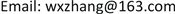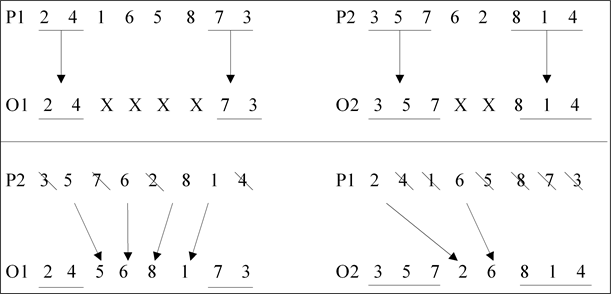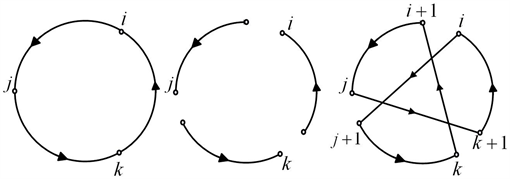﻿ 基于交叉算子和邻域搜索算子的离散粒子群优化算法 A Novel Discrete Particle Swarm Optimization Algorithm Base on Crossover Operator and Neighborhood Search

Computer Science and Application
Vol.07 No.12(2017), Article ID:23212,8 pages
10.12677/CSA.2017.712141

A Novel Discrete Particle Swarm Optimization Algorithm Base on Crossover Operator and Neighborhood Search

Wenxue Zhang1, Xiaoning Wei2, Xiaowei Wan3

1School of Sciences, Ningxia Medical University, Yinchuan Ningxia

2Department of Information, Affiliated Hui Medicine & Chinese Medicine Hospital to Ningxia Medical University, Wuzhong Ningxia

3Department of Information, The Fifth People’s Hospital of Ningxia, Shizuishan NingxiaReceived: Dec. 4th, 2017; accepted: Dec. 17th, 2017; published: Dec. 28th, 2017ABSTRACT

Based on crossover operator, neighborhood search and the essential mechanism of information updating in particle swarm optimization, a novel discrete particle swarm optimization (NDPSO) algorithm is proposed in which some basic operations on particles velocity and location are redefined. The NDPSO is a general-purpose optimizing model for combinatorial optimization problem; It is evaluated with 23 benchmark instances of flowshop scheduling problem and found to be more efficient and effective than existing algorithms.

Keywords:Discrete Particle Swarm Optimization, Crossover Operator, Neighborhood Search, Combinatorial Optimization, Flowshop Scheduling

1宁夏医科大学 理学院，宁夏 银川

2宁夏医科大学附属回医中医医院 信息科，宁夏 吴忠

3宁夏第五人民医院 信息科，宁夏 石嘴山1. 引言

2. 标准PSO

Eberhard和Kennedy于1995年提出PSO后，该算法得到各领域学者的广泛研究。为了更好的控制算法寻优能力，1998年Shi等  进行了具有里程碑意义的研究，提出了惯性权重 $\omega$ ，用 $\omega$ 来控制速度变化，较大的 $\omega$ 可以加强PSO的全局搜索能力，较小的 $\omega$ 则能加强局部搜索能力。Shi等引入惯性权重的PSO被诸多学者称为标准PSO算法，可描述如下：

${v}_{id}\left(t+1\right)=\omega {v}_{id}\left(t\right)+{c}_{1}\xi \left({p}_{id}\left(t\right)-{x}_{id}\left(t\right)\right)+{c}_{2}\eta \left({p}_{gd}\left(t\right)-{x}_{id}\left(t\right)\right)$ (1)

${x}_{id}\left(t+1\right)={v}_{id}\left(t+1\right)+{x}_{id}\left(t\right),i=1,2,\cdots ,m,$ (2)

${P}_{i}\left(t+1\right)=\left\{\begin{array}{l}{X}_{i}\left(t+1\right),\text{ }\text{ }\text{ }\text{if}\text{ }f\left({X}_{i}\left(t+1\right)\right) (3)

The procedure of PSO is given as follows:

Step 1: Initialization

Step 1.1. Initialize iterative counter be $t=0$ , the $m$ random velocities ${v}_{i}\left(0\right)$ and the $m$ random positions ${x}_{i}\left(0\right)$ of the particles;

Step 1.2. Calculate ${p}_{i}\left(0\right)$ and ${p}_{g}\left(0\right)$ ;

Step 2: While termination criteria is satisfied do

Step 2.1. for ( $i=1;i<=m;i++$ ) {

Update ${v}_{i}\left(t\right)$ using (1);

Update ${x}_{i}\left(t\right)$ using (2);

Update ${p}_{i}\left(t\right)$ using (3);

If ( $f\left({p}_{i}\left(t\right)\right) ) then ${p}_{g}\left(t\right)={p}_{i}\left(t\right)$ ;

}//end for

Step 2.2. $t=t+1$ ;

Step 3: output.

3. 新型离散粒子群优化(NDPSO)

3.1. 粒子位置与位置的减法及速度数乘的复合运算

Murata等  提了置换流水车间调度问题最好的交叉算子(crossover for permutation flowshop scheduling, CPFS)：按交叉概率随机地从种群中选择两个个体作为父体，对于每个个体选取最先与最后回溯的工件位置作为交叉点，如果回溯工件个数小于(或等于) 1，则随机选择某两个(或一个)工件位置作为交叉点。首先将两个位置之前和之后的基因进行交叉复制，再按照父体中原来工件排列的顺序修补交叉部分中未包含的基因，该交叉操作既可以尽可能多地保留没有发生回溯的工件位置信息，又可以继承父染色体中工件之间的相对位置信息。如图1所示。

3.2. 常数与速度的乘法Figure 1. CPFS process diagramFigure 2. Insert(x, y) process diagramFigure 3. Swap(x, y) process diagram

Step 1：生成 $\left[0,1\right]$ 之间的随机数 $rand\left(\text{ }\right)$$\left[1,n\right]$ 之间的随机整数 $randInt\left(\text{ }\right)$ ，令 $t=0$${V}^{\prime }=V$

Step 2： $t++$ ; if $t\le randInt\left(\text{ }\right)$ , then go to Step 3; otherwise, go to Step 4；

Step 3：if $rand\left(\text{ }\right)\le c$ , then以 $V$ 为初始解，随机选取邻域搜索算子Swap(x, y)、Insert(x, y)和PD3opt进行邻域搜索，并根据目标函数值更新 ${V}^{\prime }$ ；otherwise, go to Step 4；

Step 4：stop, output.

3.3. 速度与速度加法的复合运算

3.4. 速度与位置的加法运算

3.5. NDPSO中粒子速度和位置的更新公式Figure 4. PD3opt process diagram

${V}_{t}^{p}={c}_{1}×\left({X}^{p}-{X}_{t}\right)$ (4)

${V}_{t}^{g}={c}_{2}×\left({X}^{g}-{X}_{t}\right)$ (5)

${V}_{t+1}=\left(\omega \cdot {V}_{t}\right)+{V}_{t}^{p}+{V}_{t}^{g}$ (6)

${X}_{t+1}={X}_{t}\oplus {V}_{t+1}$ (7)

4. 仿真实验

4.1. 实验设计和参照算法

NDPSO算法的基本流程与标准PSO算法相同，只是根据公式(4)、(5)、(6)和(7)更新种群。种群大小为100，最大迭代次数为500。每组实验独立运行30次，记录makespan 和CPU的平均值。

4.2. 实验结果与分析Table 1. Comparison of the experiment results obtained by various algorithms

1) 在求解质量方面，本文提出的NDPSO算法明显优于其它算法。这表明本文基于粒子群基本优化机制的NDPSO算法具有良好的求解性能，可用于组合优化问题的求解。

2) 在计算时间方面，本文提出的NDPSO算法略差于DS+M算法、TS + M算法和DPSO算法，但明显优于VNS和GASA算法。

5. 结束语

A Novel Discrete Particle Swarm Optimization Algorithm Base on Crossover Operator and Neighborhood Search[J]. 计算机科学与应用, 2017, 07(12): 1262-1269. http://dx.doi.org/10.12677/CSA.2017.712141

1. 1. Eberhard, R. and Kennedy, J. (1995) A New Optimizer Using Particle Swarm Theory. Proceedings of Sixth International Symposium on Micro Machine and Human Science, Nagoya, 4-6 October 1995, 39-43. https://doi.org/10.1109/MHS.1995.494215

2. 2. Kennedy, J. and Eberhard, R. (1995) Particle Swarm Optimization. Proceedings of IEEE Conference on Neural Networks, Piscataway, IEEE Press, 1942-1948. https://doi.org/10.1109/ICNN.1995.488968

3. 3. Lin, C.J. and Hsieh, M.H. (2008) Classification of Mental Task from EEG Data Using Neural Networks Based on Particle Swarm Optimization. Neurocomputing, 72, 1121-1130. https://doi.org/10.1016/j.neucom.2008.02.017

4. 4. 谈超, 李小平. 双目标无等待流水线调度的加权混合算法[J]. 计算机科学, 2008(11): 199-213.

5. 5. Karakuzu, C. (2008) Fuzzy Controller Training Using Particle Swarm Optimization for Nonlinear System Control. ISA Transactions, 47, 229-239. https://doi.org/10.1016/j.isatra.2007.09.003

6. 6. 沈林成, 霍霄华, 牛轶峰. 离散粒子群优化算法研究现状综述[J]. 系统工程与电子技术, 2008(10): 1986-1994.

7. 7. Clerc, M. (2004) Discrete Particle Swarm Optimization Illustrated by the Traveling Salesman Problem. Springer Berlin Heidelberg, 47, 219-239. https://doi.org/10.1007/978-3-540-39930-8_8

8. 8. Wang, K.P., Huang, L., Zhou, C.G., et al. (2003) Particle Swarm Optimization for Traveling Salesman Problem. International Conference on Machine Learning and Cybernetics, 3, 1583-1585.

9. 9. Shi, X.H., Liang, Y.C., Lee, H.P., et al. (2007) Particle Swarm Optimization-Based Algorithms for TSP and Generalized TSP. Information Processing Letters, 103, 169-176. https://doi.org/10.1016/j.ipl.2007.03.010

10. 10. Shi, Y. and Eberhard, R. (1998) A Modified Particle Swarm Optimizer. IEEE World Congress on Computational Intelligence, Anchorage, 69-73. https://doi.org/10.1109/ICEC.1998.699146

11. 11. Murata, T., Ishibuchi, H. and Tanaka, H. (1996) Genetic Algorithms for Flowshop Scheduling Problems. Computers and Industrial Engineering, 30, 1061-1071. https://doi.org/10.1016/0360-8352(96)00053-8

12. 12. Eksioglu, B., Eksioglu, S.D. and Jain, P. (2008) A Tabu Search Algorithm for the Flowshop Scheduling Problem with Changing Neighborhoods. Computers & Industrial Engineering, 54, 1-11. https://doi.org/10.1016/j.cie.2007.04.004

13. 13. Lawler, E.L., Lenstra, J.K., Rinnooy, K.A., et al. (1997) The Traveling Salesman Problem—A Guided Tour of Combinatorial Optimization. John Wiley & Sons, Hoboken.

14. 14. Grabowski, J. and Pempera, J. (2005) Some Local Search Algorithm Search for No-Wait Flow-Shop Problem with Makespan Criterion. Computers & Operations Research, 32, 2197-2212. https://doi.org/10.1016/j.cor.2004.02.009

15. 15. Schuster, C.J. and Framinan, J.M. (2003) Approximative Procedures for No-Wait Job Shop Scheduling. Operations Research Letters, 31, 308-318. https://doi.org/10.1016/S0167-6377(03)00005-1

16. 16. 潘全科, 谢圣献, 张亚卿, 等. 解决无等待流水线调度问题的新算法[J]. 机械科学与技术, 2006(12): 1487-1490.

17. 17. Rajendran, C. (1994) A No-Wait Flowshop Scheduling Heuristic to Minimize Makespan. Journal of the Operational Research Society, 45, 472-478. https://doi.org/10.1057/jors.1994.65